## Addressing Modes of 80386 Microprocessor:

The 80386 can operate in all the addressing modes which were available with the 80286 processor. The 80386 processor can also operate in all addressing modes of 80286 with 32-bit immediate or 32-bit register operands or displacements. Besides all addressing modes of 80286, the 80386 has a family of scaled modes. In the scaled modes, the index register values will be multiplied by a valid scale factor to get the final displacement. The valid scale factors are 1, 2, 4 and 8. In this section, all scaled modes are briefly explained.

Scaled Indexed Mode The content of an index register is multiplied by a scale factor 1, 2, 4 or 8 and subsequently the computed value will be added to get the final operand offset. For example,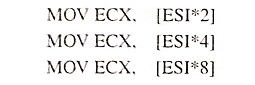Based Scaled Indexed Mode The based scaled indexed mode instruction is the content of an index register is multiplied by a scale factor and the computed value is added with the base register to find the offset.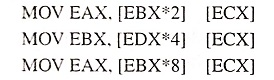Based Scaled indexed Mode with Displacement The based scaled indexed mode with displacement instruction is the content of an index register multiplied by a scaling factor and the computed value is added with the content of base register and a displacement to obtain the address of an operand. The offset can be expressed as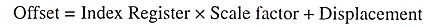and the 32-bit memory address is computed by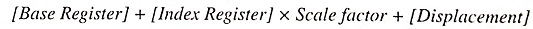The above expression can be expressed for different registers as given below: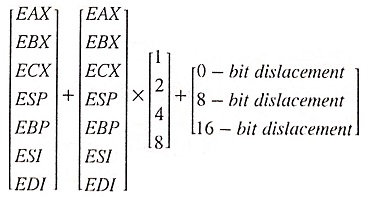The example of based scaled indexed mode with displacement instructions are

Scroll to Top